# Suppose the joint density of (X, Y ) is: fX,Y (u, v) = u + v...

Suppose the joint density of (X, Y ) is: fX,Y (u, v) = u + v for 0 ≤ u, v ≤ 1, and 0 otherwise. Compute the marginal density of X. compute E(X) and Var(X)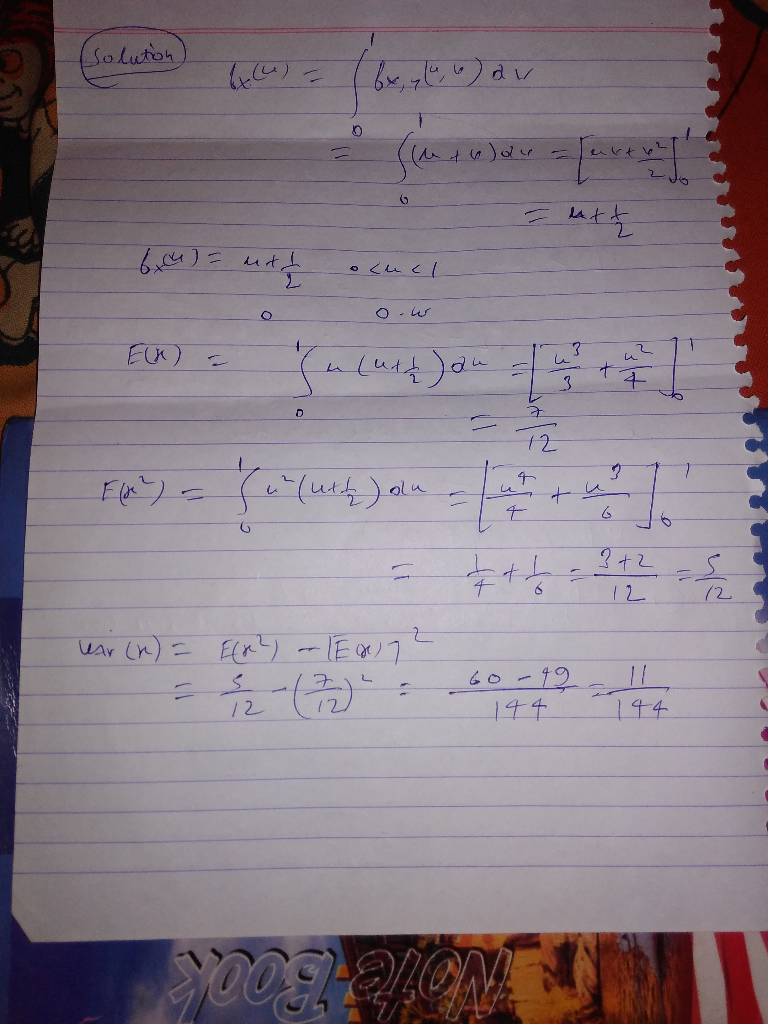#### Earn Coin

Coins can be redeemed for fabulous gifts.

Similar Homework Help Questions
• ### Suppose X and Y are jointly continuous random variables with joint density function Let U =...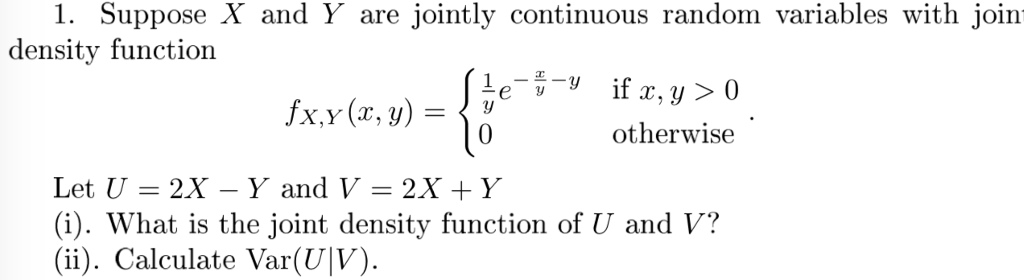Suppose X and Y are jointly continuous random variables with joint density function Let U = 2X − Y and V = 2X + Y (i). What is the joint density function of U and V ? (ii). Calculate Var(U |V ). 1. Suppose X and Y are jointly continuous random variables with join density function Lei otherwise Let U = 2X-Y and V = 2X + y (i). What is the joint density function of U and V? (ii)....

• ### Q.4 (22') Suppose the joint probability density function of X and Y is fx,y(x, y) =...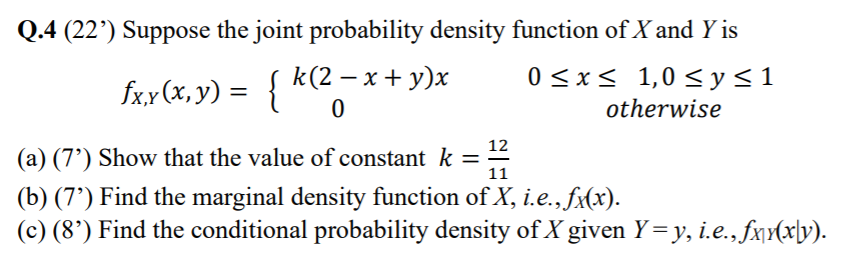Q.4 (22') Suppose the joint probability density function of X and Y is fx,y(x, y) = { „) - k(2 - x + y)x 0 sxs 1,0 sys1 o otherwise (a) (7”) Show that the value of constant k = 12 (b) (7') Find the marginal density function of X, i.e., fx(x). (c) (8') Find the conditional probability density of X given Y=y, i.e., fxy(xly). 11

• ### Suppose X and Y have joint probability density function fX,Y(x,y)=70e?3x?7y for 0<x<y; and fX,Y(x,y)=0 otherwise. Find...

Suppose X and Y have joint probability density function fX,Y(x,y)=70e?3x?7y for 0<x<y; and fX,Y(x,y)=0 otherwise. Find E(X). (You may either use the joint density given here,

• ### 1. Suppose X and Y are jointly continuous random variables with joint density function otherwise Let...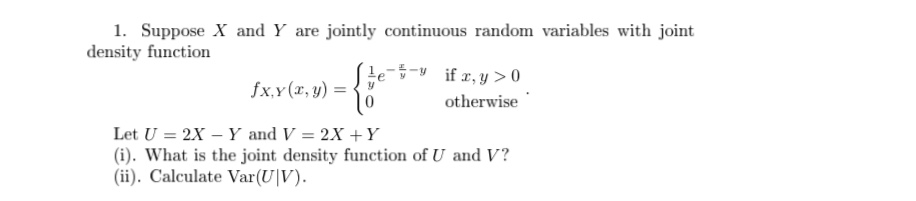1. Suppose X and Y are jointly continuous random variables with joint density function otherwise Let U 2X-Y and V-2X +Y (i). What is the joint density function of U and V? (ii). Caleulate Var(UV)

• ### 1. Let X and Y be continuous random variables with joint pr ability density function 6e2re5y İfy < 0 and x < otherwise. y, fx,y (z,y) 0 (a) [3 points] Show that the marginal density function...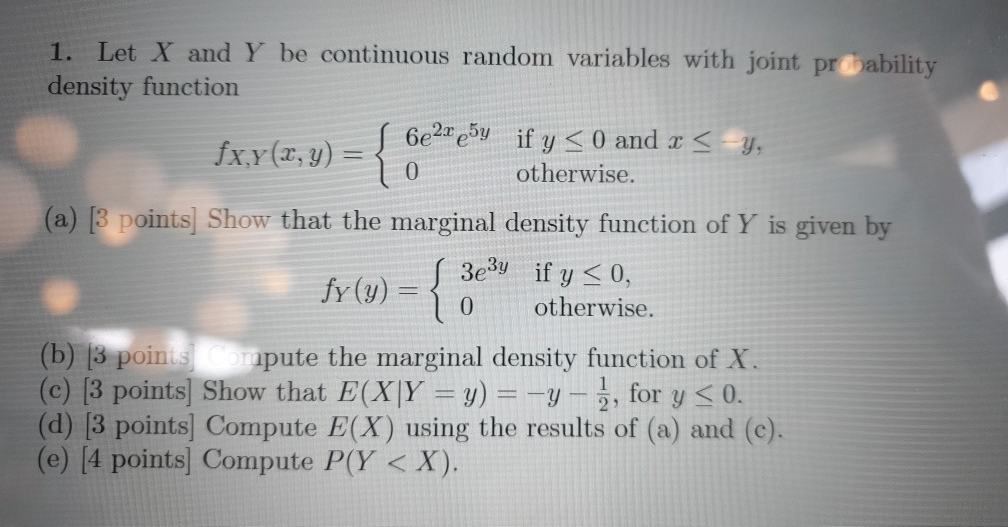1. Let X and Y be continuous random variables with joint pr ability density function 6e2re5y İfy < 0 and x < otherwise. y, fx,y (z,y) 0 (a) [3 points] Show that the marginal density function of Y is given by 3es if y 0, 0 otherwise. fy (y) = (b) |3 poin s apute the marginal density function of X (c) [3 points] Show that E(X)Y = y) =-y-1, for y 0 (d) 13 points] Compute E(X) using the...

• ### 2.9.10 Suppose X has density fX(x) = x3/4 for 0 < x < 2, otherwise fx(x)...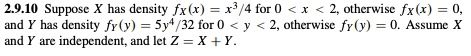2.9.10 Suppose X has density fX(x) = x3/4 for 0 < x < 2, otherwise fx(x) = 0, and Y has density fr (y)-5y4/32 for 0 < y < 2, otherwise fr (y)-0. Assume X and Y are independent, and let Z = X + Y (a) Compute the joint density fx.r(x. y) for all x, y e R (b) Compute the density fz(z) for 2.

• ### 1. Suppose X and Y are jointly continuous random variables with joint density function otherwise Let...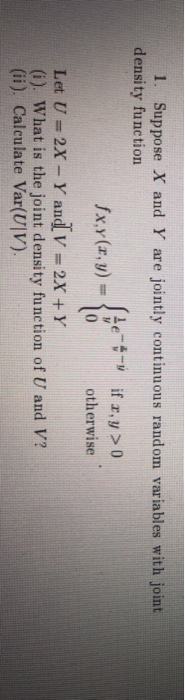1. Suppose X and Y are jointly continuous random variables with joint density function otherwise Let u=2x-Yand, V = 2X + Y (i). What is the joint density function of U and V? (ii). Calculate Var(UIV).

• ### 2.8.14 Let X and Y have joint density fX,Y (x, y) = (x2 + y)/36 for...

2.8.14 Let X and Y have joint density fX,Y (x, y) = (x2 + y)/36 for −2 < x < 1 and 0 < y < 4, otherwise fX,Y (x, y) = 0. (a) Compute the conditional density fY|X (y|x) for all x, y ∈ R1 with fX (x) > 0. (b) Compute the conditional density fX|Y (x|y) for all x, y ∈ R1 with fY (y) > 0. (c) Are X and Y independent? Why or why not?

• ### 1. Consider two random variables X and Y with joint density function f(x, y)-(12xy(1-y) 0<x<1,0<p<1 otherwise...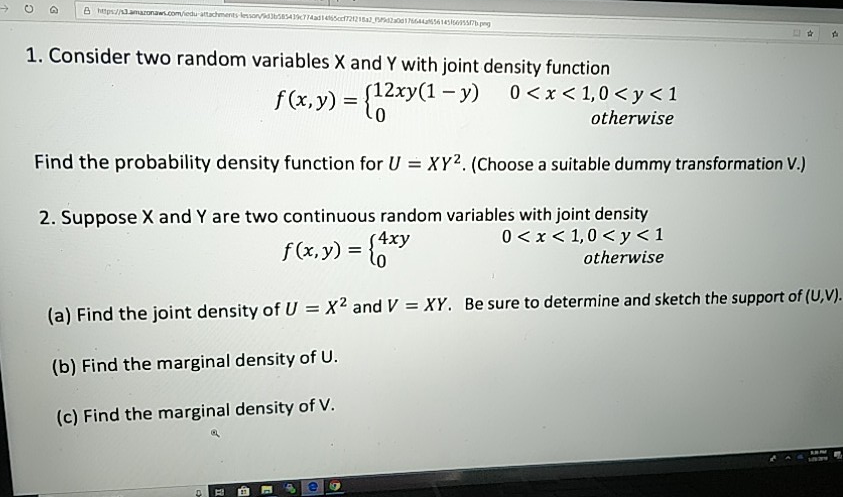1. Consider two random variables X and Y with joint density function f(x, y)-(12xy(1-y) 0<x<1,0<p<1 otherwise 0 Find the probability density function for UXY2. (Choose a suitable dummy transformation V) 2. Suppose X and Y are two continuous random variables with joint density 0<x<I, 0 < y < 1 otherwise (a) Find the joint density of U X2 and V XY. Be sure to determine and sketch the support of (U.V). (b) Find the marginal density of U. (c) Find...

• ### Let X, Y be jointly continuous with joint density function (pdf) fx,y(x, y) *(1+xy) 05 x...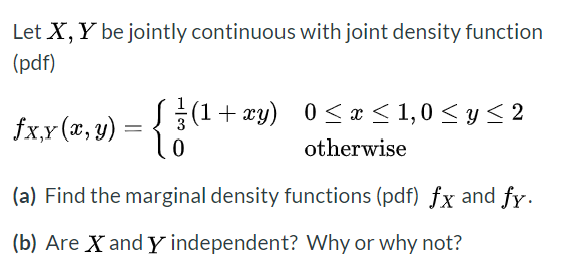Let X, Y be jointly continuous with joint density function (pdf) fx,y(x, y) *(1+xy) 05 x <1,0 <2 0 otherwise (a) Find the marginal density functions (pdf) fx and fy. (b) Are X and Y independent? Why or why not?